EA request - page 21038

yes BETA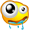Trading Hours is ALPARI Time !!!! (GTM+1)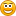#define MAGIC 20051021

extern int SLIPPAGE = 3;

extern double Lots = 0.1;

extern int StopLoss = 10;

extern int TakeProfit = 35;

extern int JPYpair = 0;

extern bool useProfitTrailing = False;

extern int TrailingStop = 20;

extern int TrailingStep = 3;

extern string PARAMETERS_EXPERT = "PARAMETERS EXPERT";

extern bool UseOneAccount = False;

extern int NumberAccount = 0815;

extern string Name_Expert = "BETA";

extern bool UseSound = True;

extern string NameFileSound = "expert.wav";

extern color clOpenSell = LightCoral;

extern color clModifySell = Tomato;

extern color clCloseSell = Red;

int prevBar;

void SetArrow(datetime t, double p, int k, color c) {

ObjectSet("Arrow", OBJPROP_TIME1 , t);

ObjectSet("Arrow", OBJPROP_PRICE1 , p);

ObjectSet("Arrow", OBJPROP_ARROWCODE, k);

ObjectSet("Arrow", OBJPROP_COLOR , c);

}

void deinit()

{

Comment("");

}

void start() {

SetArrow(Time,Low-5*Point,241,Gold);

//bx=False;

//sx=False;

Comment("");

if (UseOneAccount && AccountNumber()!=NumberAccount) {

Comment("-UseOnAccount- Number"+AccountNumber()+" FAILED!");

return;

} else Comment("");

CheckForOpen();

CheckForClose();

if (useProfitTrailing) TrailingPositions();

}

void CheckForOpen() {

double ldStop=0, ldTake=0;

double close1=iClose(NULL,1440,1);

double high1=iHigh(NULL,1440,1);

double low1=iLow(NULL,1440,1);

double close=iClose(NULL,1440,0);

double high=iHigh(NULL,1440,0);

double low=iLow(NULL,1440,0);

double Pr = high1 + 1*Point;

double Pr2 = low1 - 1*Point;

if (JPYpair == 0)

double val2 = (Pr - (MathFloor(close1*100) /100))*10000;

else

val2 = (Pr - MathFloor(close1))*100;

if (val2 >= 15 && val2 <= 85) tradesignal2 = 1;

if (JPYpair == 0)

double val2a = (Pr2 - (MathFloor(close1*100) /100))*10000;

else

val2a = (Pr2 - MathFloor(close1))*100;

if (val2a >= 15 && val2a <= 85) tradesignal2s = 1;

int T=0;

if(Hour()== 23 && Minute() == 30) T=1;

if (!ExistPosition() && prevBar!=Bars) {

if (T==1 && tradesignal2 == 1 && (high1 - low1) >= 100*Point && (high1 - close1 ) >= 25*Point ) {

if (StopLoss!=0) ldStop=Pr-StopLoss*Point;

if (TakeProfit!=0) ldTake=Pr+TakeProfit*Point;

prevBar=Bars;

}

if (T== 1 && tradesignal2s == 1 && (high1 - low1) >= 100*Point && (close1 - low1) >= 25*Point ) {

if (StopLoss!=0) ldStop=Pr2+StopLoss*Point;

if (TakeProfit!=0) ldTake=Pr2-TakeProfit*Point;

SetOrder(OP_SELLSTOP, Pr2, ldStop, ldTake);

prevBar=Bars;

}

}

}

//+------------------------------------------------------------------+

//| is MAGIC trade open ? |

//+------------------------------------------------------------------+

bool ExistPosition() {

bool Exist=False;

for (int i=0; i<OrdersTotal(); i++) {

if (OrderSymbol()==Symbol() && OrderMagicNumber()==MAGIC) Exist=True;

}

}

return(Exist);

}

void SetOrder(int op, double pp, double ldStop, double ldTake) {

color clOpen;

string lsComm=GetCommentForOrder();

OrderSend(Symbol(),op,Lots,pp,SLIPPAGE,ldStop,ldTake,lsComm,MAGIC,0,clOpen);

if (UseSound) PlaySound(NameFileSound);

}

string GetCommentForOrder() {

return(Name_Expert);

}

void TrailingPositions() {

for (int i=0; i<OrdersTotal(); i++) {

if (OrderSymbol()==Symbol() && OrderMagicNumber()==MAGIC ) {

if ((Bid-OrderOpenPrice())>TrailingStop*Point) {

if (OrderStopLoss()<Bid-(TrailingStop+TrailingStep-1)*Point) {

}

}

}

if (OrderType()==OP_SELL) {

}

}

}

}

}

}

}

void CheckForClose() {

bool fs=False;

bool fs1=False;

int T2=0;

if(Hour()== 13 && Minute() >= 45) T2=1;

if (T2==1){

fs1 = True;

fs = True;

}

for (int i=0; i<OrdersTotal(); i++) {

if (OrderSymbol()==Symbol() && OrderMagicNumber()==MAGIC) {

OrderDelete(OrderTicket());

// OrderClose(OrderTicket(), Lots, Bid, SLIPPAGE, Aqua);

return;

}

if (OrderType()==OP_SELLSTOP && fs1) {

OrderDelete(OrderTicket());

// OrderClose(OrderTicket(), Lots, Ask, SLIPPAGE, Violet);

return;

}

}

}

}

}

void ModifyStopLoss(double ldStop, color clModify) {

bool fm;

double ldOpen=OrderOpenPrice();

double ldTake=OrderTakeProfit();

fm=OrderModify(OrderTicket(), ldOpen, ldStop, ldTake, 0, clModify);

if (fm && UseSound) PlaySound(NameFileSound);

}1038

here EAFiles:
platinum.mq4 7 kb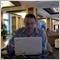Moderator
110865

Alex,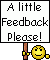Which timeframe for Platimun and Beta?8

Eric:
Sada, I think it means for example:

Do not go long if price is 1.1785 through 1.1799 (because there will be resistance at the "round" number -- 1.1800, and it might retrace back down)

Do not go short if price is 1.1715 through 1.1701 (because there will be support at the "round" number -- 1.1700, and it might retrace back up)

But I could be wrong!

eric, I think you are wrong he means

do not trade short OR long if price is 1.1685 through 1.1715 because he assumes this is a zone of great support or resistance.

xpie1038

i have auto daily D1 chart all time frame

yes xpie is all Beta help me please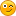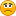307

xpie, yes it looks like you are right -- there is a 30 pip "no trade zone" for longs and shorts.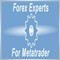4337

The similiar strategy at EasyDayTrader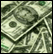is ready to use. Chek it out.466

Alex.Piech.FinGeR:
yes BETA.

can u send meh the gso? you can email it if u like to forextrash<--yahoo710

forex-experts:
The similiar strategy at EasyDayTraderis ready to use. Chek it out.

Maybe you could post an EXP or EX4 trial version (30 days?)of the EA for us to test drive?

Otherwise, one might think you are posting here just to advertise your commercial EAs and not for the benefit of the forum members...that is call free spamming,right? Now, we don't want that do we?Here is a link to where I think your future "advertizing" post should go (commercial EA) :

https://www.mql5.com/en/forum/173061

Edit:

PS: my post,after reading it, sounds a little bit too sarcastic...sorry if I have offended you but I am actually really interested in testing out commercial EAs.3

Hello,

Is anybody still trading/interested in this system, and are you having success?

I downloaded and compiled the indicator source and it does show up in MT4's "Custom" menu but nothing happens when I select the indicator. Strange.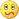Update: Ah, I see--it's an expert and not an indicator. My mistake. Still curious about anyone's current opinions of this system!